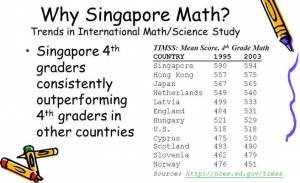Jeff Bezos teaches his kids math with a strategy that’s made Chinese and Singaporean students the best in the world — but it’s unusual in the US

Singapore math, also known as the “mastery approach,” is a method of teaching mathematics that’s most prevalent in many Asian countries. Under the mastery approach, students learn a specific concept before moving on to more complex ideas, in a rigidly linear progression. Students aren’t grouped by their perceived intellectual abilities. Instead, all students perform the same work at the same time before mastering the concept and advancing to the next one together.

By contrast, US schools teach math using the “mindset approach,” which aims to teach students a more intuitive understanding of math concepts by starting with a broader concept before breaking down a problem into the specific steps for solving.

#### WHAT OUR PARENTS SAY?

MICS Singapore math class helps give children confidence in math, in a fun, yet challenging environment. We have been recommending the program to friends for many years and will continue to do so.

My daughter developed a lot of confidence in her math abilities as well as very solid math skills. She really enjoyed the group work (and her fun and funny classmates).

MICS Singapore math program has helped our children lay a very strong foundation in mathematics. Not only have they learned the various concepts in mathematics but they have also improved their problem-solving skills.”

The classes are interesting and motivating. My daughter loves that there are high expectations of her and that she really has to think hard to be successful.

#### CORE CURRICULUM

• Level 1
• Correctly grasp the bit and multi-digit addition and subtraction
• The number of digital means
• Learn to analyze word problems, know how to solve the problem
• On the currency and be aware of the basic three-dimensional graphics
• Level 2
• Correctly grasp the single-digit multiplication and division
• Mixed arithmetic and word problems
• Understanding of line and time
• Understand the million less than the number of pronunciation and writing
• Level 3
• Correctly grasp the double-digit multiplication and division
• Weight units and their understanding of how the conversion
• Correct analysis of two-step word problems, and can out-type solution
• Learn the basics of statistics
• Master Square, rectangular and parallelogram meaning, learning calculates the perimeter of squares and rectangles
• Level 4
• Proper understanding of multiplication and division of integers
• Arithmetic order and the correct answer
• Understanding of angles, perpendicular and parallel lines
• Understanding of fractions and decimals, and control the definition of decimal addition and subtraction operations
• Level 5
• Divisible by the number of correctly grasp
• Cuboids and the cube of the meaning, and the volume and surface area calculation
• Preliminary knowledge of statistics